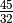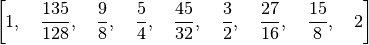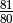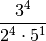# Number Theory¶

While this part of the package isn’t particularly fleshed out yet, there are a few number-theoretic functions for the analysis of scales.

## Odd Limits¶

PyTuning contains functions for finding the odd Limit for both intervals and scales.

We can define and interval – say,, and find its odd-limit with the following:

from pytuning.number_theory import odd_limit

interval = sp.Rational(45,32)
limit = odd_limit(interval)


which yields and answer of 45.

One can also find the odd limit of an entire scale with the find_odd_limit_for_scale() function:

from pytuning.scales import create_euler_fokker_scale
from pytuning.number_theory import find_odd_limit_for_scale

scale = create_euler_fokker_scale([3,5],[3,1])
limit = find_odd_limit_for_scale(scale)


which yields 135. (Examining the scale:you will see that this is the largest odd number, and is found in the second degree.)

pytuning.number_theory.odd_limit(i)

Find the odd-limit of an interval.

Parameters: i – The interval (a sympy.Rational) The odd-limit for the interval.
pytuning.number_theory.find_odd_limit_for_scale(s)

Find the odd-limit of an interval.

Parameters: s – The scale (a list of sympy.Rational values) The odd-limit for the scale.

## Prime Limits¶

One can also compute prime limits for both scales and intervals. Extending the above example, one would assume that the Euler-Fokker scale would have a prime-limit of 5, since that’s the highest prime used in the generation, and in fact:

from pytuning.scales import create_euler_fokker_scale
from pytuning.number_theory import find_prime_limit_for_scale

scale = create_euler_fokker_scale([3,5],[3,1])
limit = find_prime_limit_for_scale(scale)


will return 5 as the limit.

pytuning.number_theory.prime_limit(i)

Find the prime-limit of an interval.

Parameters: i – The interval (a sympy.Rational) The prime-limit for the interval.
pytuning.number_theory.find_prime_limit_for_scale(s)

Find the prime-limit of an interval.

Parameters: s – The scale (a list of sympy.Rational values) The prime-limit for the scale.

## Prime Factorization of Degrees¶

PyTuning has functions for breaking a ratio down into prime factors, and the inverse of reassembling them.

pytuning.number_theory.prime_factor_ratio(r, return_as_vector=False)

Decompose a ratio (degree) to prime factors

Parameters: r – The degree to factor (sympy.Rational) return_as_vector – If True, return the factors as a vector (see below) The factoring of the ratio

By default this function will return a dict, with each key being a prime, and each value being the exponent of the factorization.

As an example, the syntonic comma,, can be factored:

r = syntonic_comma
q = prime_factor_ratio(r)
print(q)
{2: -4, 3: 4, 5: -1}


which can be interpreted as:If return_as_vector is True, the function will return a tuple, with each position a successive prime. The above example yields:

r = syntonic_comma
q = prime_factor_ratio(r, return_as_vector=True)
print(q)
(-4, 4, -1)


Note that zeros will be included in the vector, thus:

r = sp.Rational(243,224)
q = prime_factor_ratio(r, return_as_vector=True)
print(q)
(-5, 5, 0, -1)
q = prime_factor_ratio(r)
print(q)
{2: -5, 3: 5, 7: -1}

pytuning.number_theory.create_ratio_from_primes(factors)

Given a prime factorization of a ratio, reconstruct the ratio. This function in the inverse of prime_factor_ratio().

Parameters: factors – The factors. Can be a dict or a tuple (see prime_factor_ratio for a discussion). The ratio (sympy value).